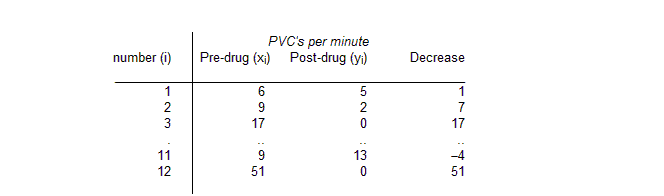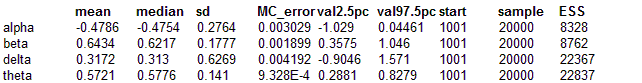#### Hearts: a mixture model for count data

The table below presents data given by Berry (1987) on the effect of a drug used to treat patients with frequent premature ventricular contractions (PVCs) of the heart.Farewell and Sprott (1988) model these data as a mixture distribution of Poisson counts in which some patients are "cured" by the drug, whilst others experience varying levels of response but remain abnormal. A zero count for the post-drug PVC may indicate a "cure", or may represent a sampling zero from a patient with a mildly abnormal PVC count. The following model thus is assumed:

xi   ~   Poisson(λi)   for all patients
yi   ~   Poisson(βλi)   for all uncured patients
P(cure)   =   θ

To eliminate nuisance parameters li, Farewell and Sprott use the conditional distribution of yi given ti = xi + yi. This is equivalent to a binomial likelihood for yi with denominator ti and probability p = b /(1+b) (see Cox and Hinkley, 1974 pp. 136-137 for further details of the conditional distribution for Poisson variables). Hence the final mixture model may be expressed as follows:

P(yi = 0 | ti )   =   θ + (1 - θ) (1 - p) ti
P(yi | ti )   =   (1 - θ) (ti! / (yi! (ti-yi)!)) (pyi (1 - p)(ti - yi)   yi = 1,2,...,ti

The BUGS code for this model is given below:

model
{
for (i in 1 : N) {
y[i] ~ dbin(P[state1[i]], t[i])
state[i] ~ dbern(theta)
state1[i] <- state[i] + 1
t[i] <- x[i] + y[i]
prop[i] <- P[state1[i]]
}
P <- p
P <- 0
logit(p) <- alpha
alpha ~ dnorm(0,1.0E-4)
beta <- exp(alpha)
logit(theta) <- delta
delta ~ dnorm(0, 1.0E-4)
}

##### Data
``` list(x = c(6, 9, 17, 22, 7, 5, 5, 14, 9, 7, 9, 51),      y = c(5, 2, 0, 0, 2, 1, 0, 0, 0, 0, 13, 0), N = 12) ```
##### Inits for chain 1
``` list(delta = 0, alpha = 0) ```

##### Inits for chain 2
``` list(delta = 2, alpha = 2) ```
Results